# Special Relativity

Student Learning Objectives
Lessons / Lecture Notes
Important Equations
Example Problems
Applets and Animations

Student Learning Objectives

• To understand the two postulates of special relativity.
• To understand how the principle of relativity leads to time dilation and length contraction.
• To understand and reason with the fundamental concepts of event simultaneity and reference frames.
• To explore relativistic energy and momentum.
• To recognize the significance of Einstein’s famous equation E = mc2.

Lessons / Lecture Notes

PY106 Notes from Boston University (algebra-based):

Physics 2C notes from Dr. Bobby W.S. Lau (algebra-based)

HyperPhysics (calculus-based)

Physics 4B notes from Dr. Bobby W.S. Lau (calculus-based)

Important Equations (for algebra-based Physics)

Example Problems

Example Problems for algebra-based physics (from Physics 8th Edition by Cutnell and Johnson):

Example Problems (Special Relativity)
Solutions to Example Problems (Special Relativity)

Applets and Animations
 Michelson-Morely Experiment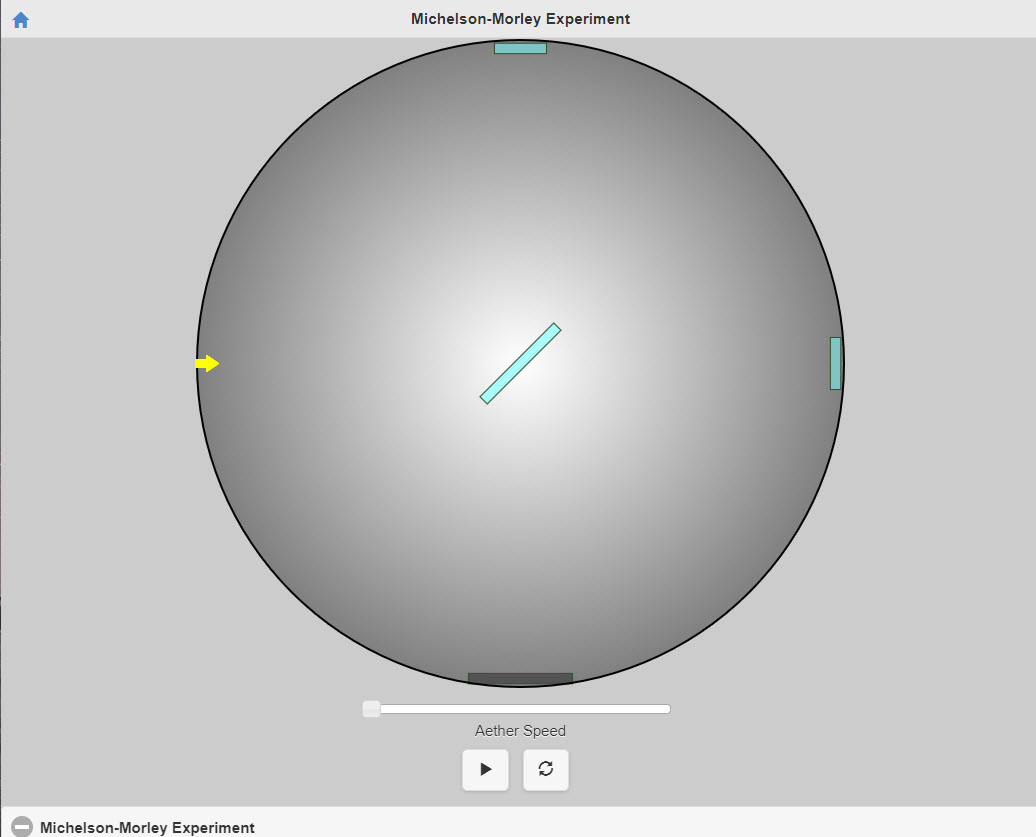This movie simulates the set-up used in the Michelson-Morley experiment, including the non-existent aether wind they were trying to detect! The basic idea is to detect the time difference between light going "upstream then downstream" and light going "across shore and back." Rotating the apparatus exchanges these two routes: you can rotate it by touch, or with a mouse. Michelson-Morley Experiment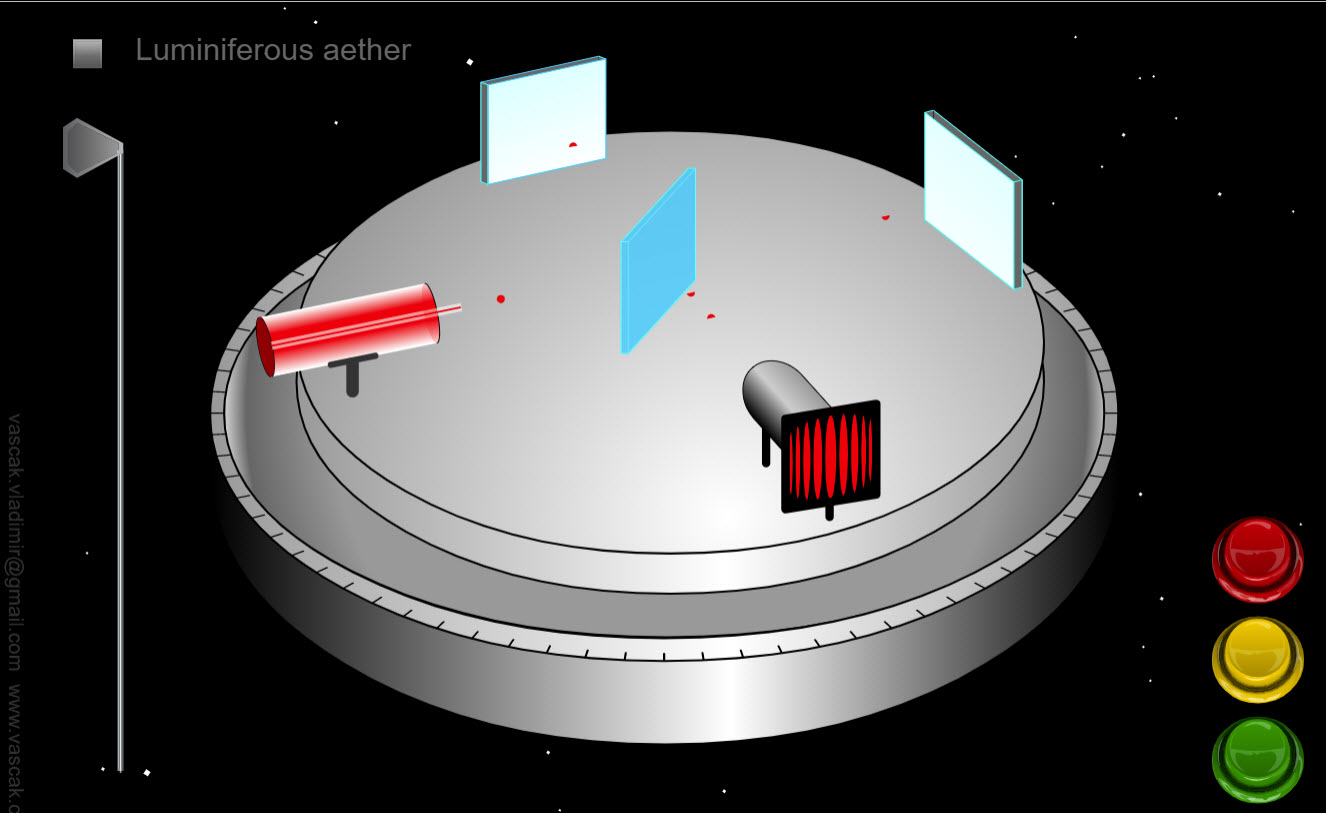This applet shows the set-up for the Michelson-Morley Experiment. There are no directions so it is a bit challenging to use this applet. Light Clock - Time Dilation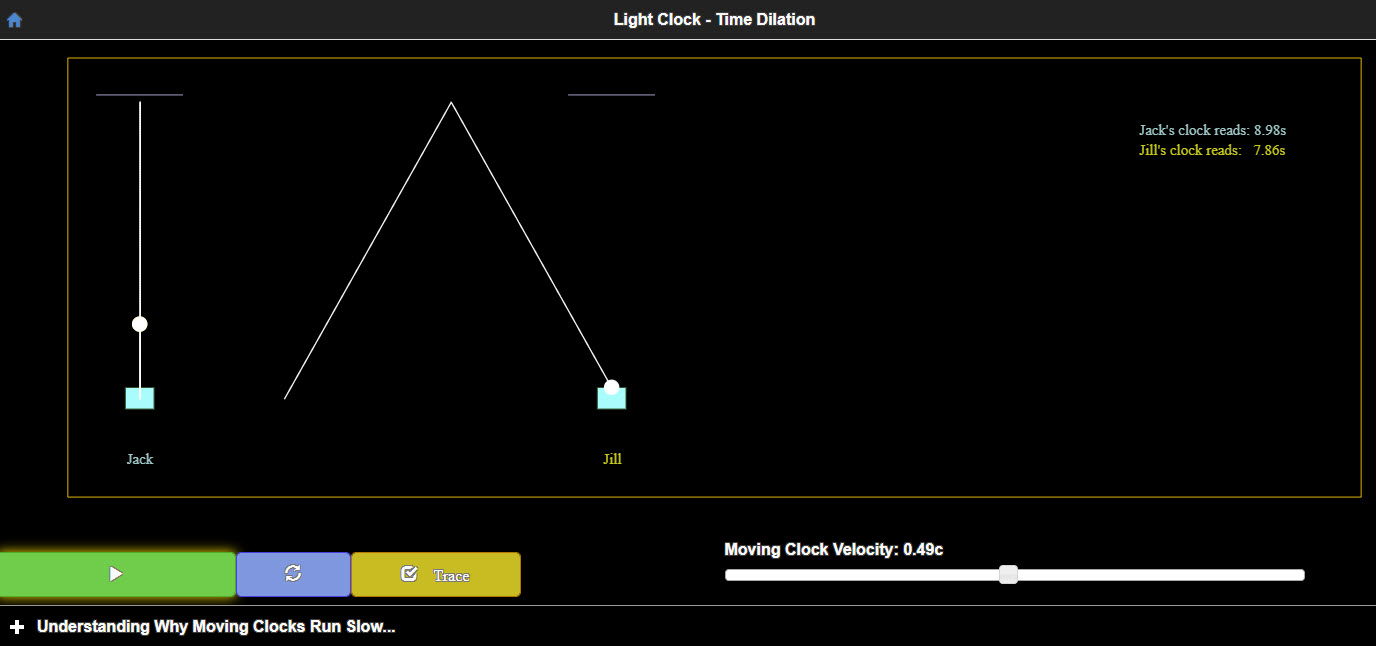Understanding why moving clocks run slow. Einstein's Special Relativity states that the laws of physics are the same in all inertial frames, specifically the same on a steadily moving train as they are on the ground. But the laws predict that the speed of light, called c, has a precise value, close to three hundred thousand kilometers per second. This means two different observers, looking at the same moving blob of light, will say that's how fast it's moving relative to them, even though they are moving relative to each other! A major consequence of this apparent paradox is that time flows at different rates in the two frames: a moving clock is observed to run slow. Here's a primitive clock: a blob of light bouncing back and forth between two fixed mirrors. If the clock is moving relative to us, the light must go further -- but its speed must be the same, from the theory, so it runs slow. The reality of this effect is well illustrated by the GPS, which depends on very accurate clocks in moving satellites. The system yields seriously incorrect results if the relativistic clock slowing is ignored. An Example of Time Dilation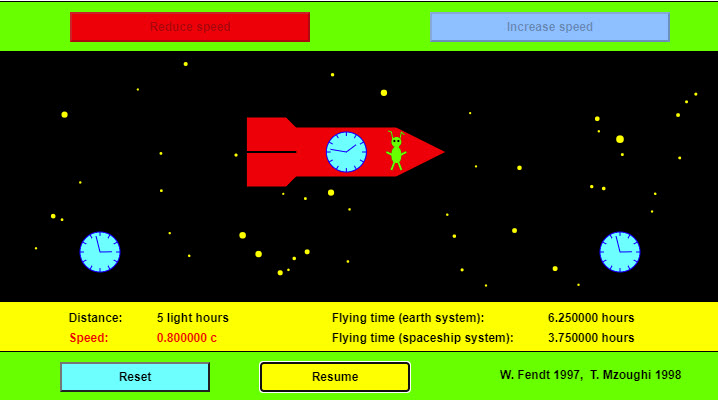A spaceship is flying a distance of 5 light hours, for example from Earth to the dwarf planet Pluto. The speed can be regulated with the upper buttons. The app demonstrates that the clock in the spaceship goes more slowly than the two clocks of the system in which Earth and Pluto are motionless. Time Dilation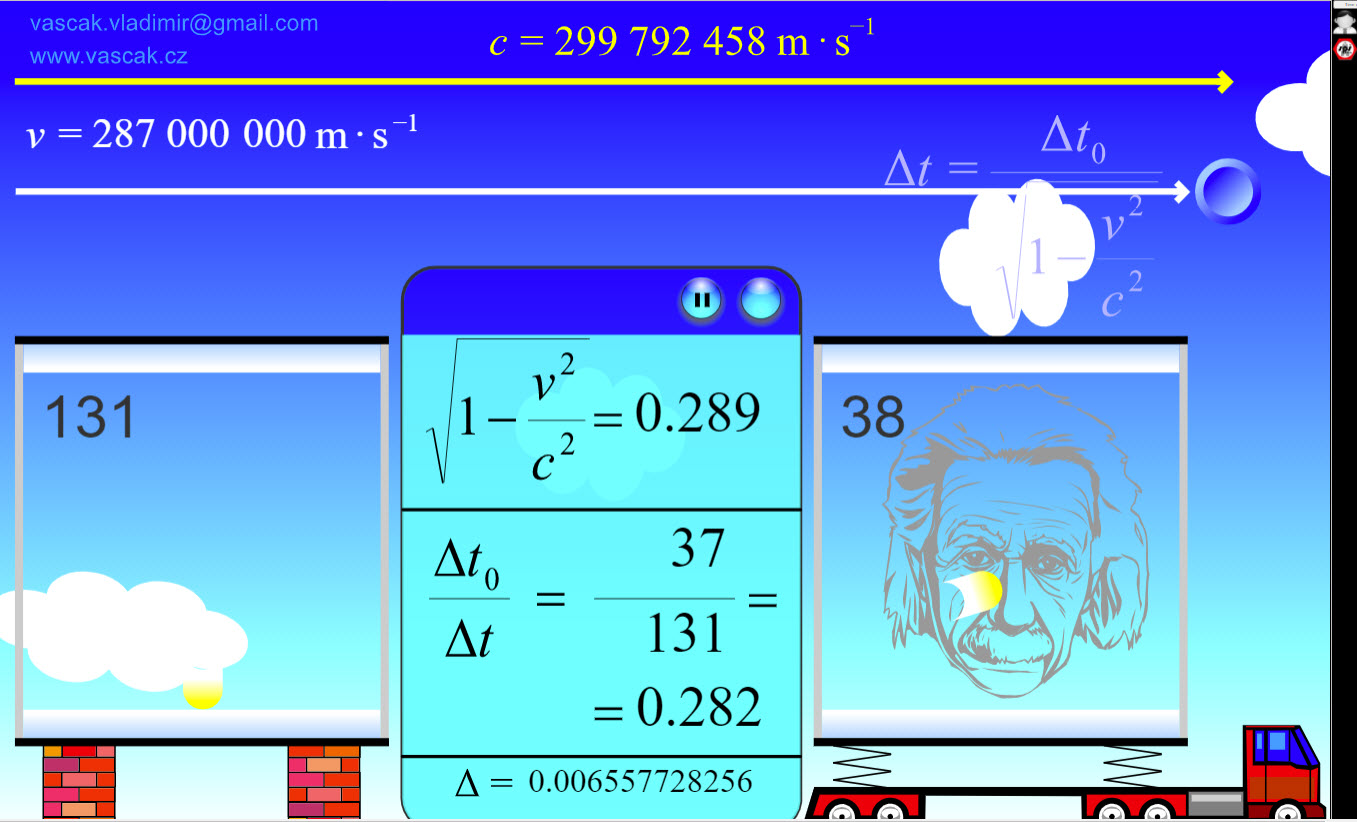This applet shows time dilation for different speeds. There are no directions so it is a bit challenging to use this applet. Length Contraction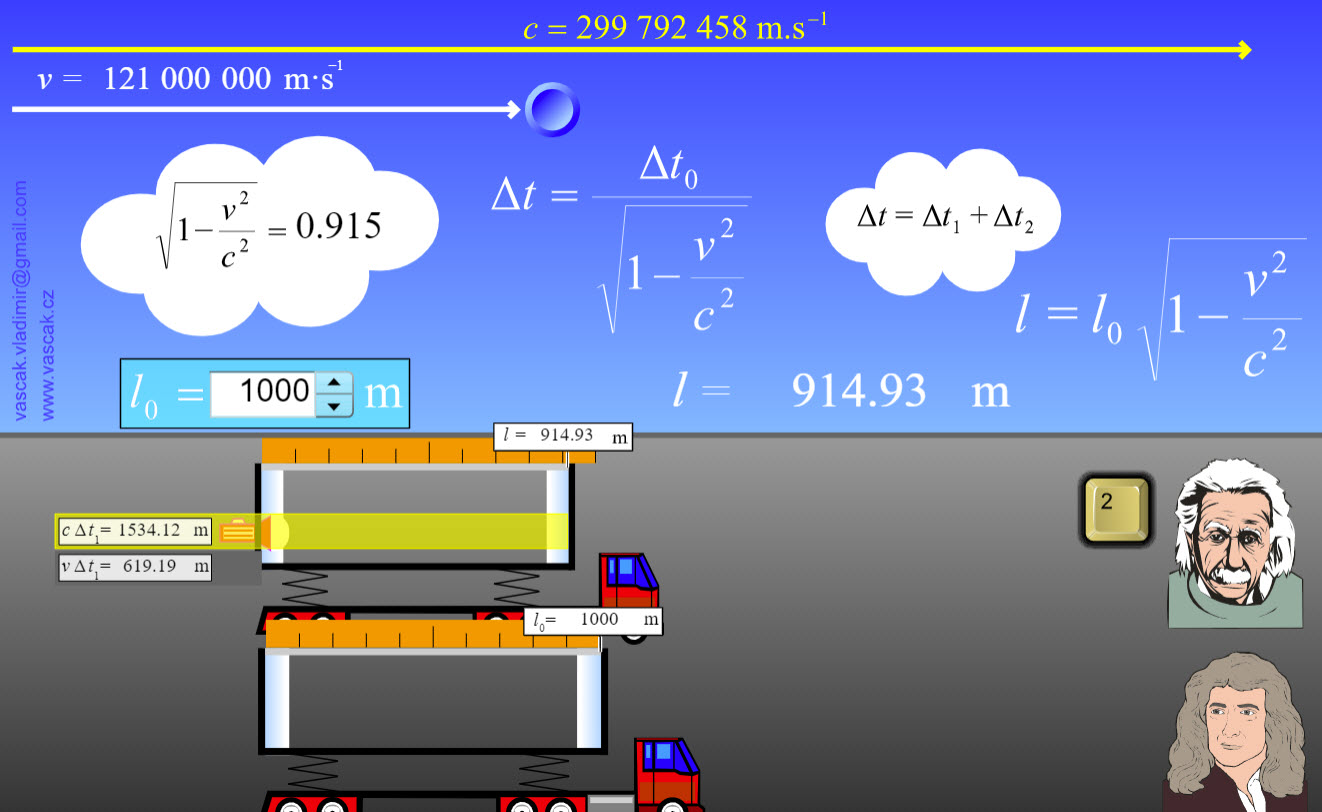This applet shows length contraction for different speeds. There are no directions so it is a bit challenging to use this applet.